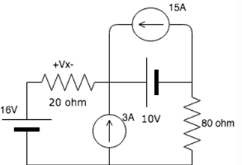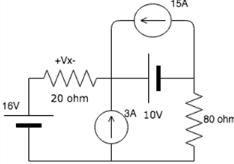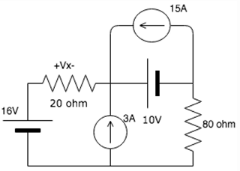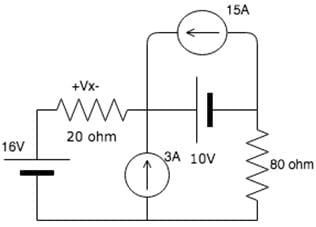# Test: Superposition Theorem

## 10 Questions MCQ Test Network Theory (Electric Circuits) | Test: Superposition Theorem

Description
Attempt Test: Superposition Theorem | 10 questions in 10 minutes | Mock test for Electrical Engineering (EE) preparation | Free important questions MCQ to study Network Theory (Electric Circuits) for Electrical Engineering (EE) Exam | Download free PDF with solutions
QUESTION: 1

### In superposition theorem, when we consider the effect of one voltage source, all the other voltage sources are____________

Solution:

In superposition theorem when we consider the effect of one voltage source, all the other voltage sources are shorted and current sources are opened.

QUESTION: 2

### In superposition theorem, when we consider the effect of one current source, all the other voltage sources are____________

Solution:

In superposition theorem, whether we consider the effect of a voltage or current source, voltage sources are always shorted and current sources are always opened.

QUESTION: 3

### In superposition theorem, when we consider the effect of one voltage source, all the other current sources are____________

Solution:

In superposition theorem when we consider the effect of one voltage source, all the other current sources are opened and voltage sources are shorted.

QUESTION: 4

In superposition theorem, when we consider the effect of one current source, all the other current sources are____________

Solution:

In superposition theorem, whether we consider the effect of a voltage or current source, current sources are always opened and voltage sources are always shorted.

QUESTION: 5

Find the value of Vx due to the 16V source.​Solution:

When we consider the 16V source, we short the 10V source and open the 15A and 3A source. From the resulting series circuit we can use voltage divider to find Vx.
Vx= 16*20/(20+80)=3.2A.

QUESTION: 6

Find Vx due to the 3A source.​Solution:

Due to the 3A source, we short the 16V and 10V source and open the 15A source. From the resulting circuit, we can use current divider to find the current in the 20 ohm branch and then multiply it with the resistance to find the voltage.
I20= 3*80/(20+80)=2.4A
Vx=20*2.4=48V.

QUESTION: 7

Find the value of Vx due to the 10V source.​Solution:

Due to the effect of the 10V source, we short the 16V source and open the 3A and 15A source. From the resulting series circuit, we can use voltage divider to find the value of Vx.
Vx=10*20/(80+20)=2V.

QUESTION: 8

Find the voltage due to the 15A source.Solution:

Due to the 15V source, the 10V and 16V sources get shorted and the 3A source acts as an open circuit. Since the 10V source is shorted, it acts as a low resistance path and current flows only within that loop and do not flow to the 20 ohm resistor. Hence the voltage is 0V.

QUESTION: 9

Superposition theorem is valid for_________

Solution:

Superposition theorem is valid only for linear systems because the effect of a single source cannot be individually calculated in a non-linear system.

QUESTION: 10

Superposition theorem does not work for________

Solution:

Power across an element is not equal to the power across it due to all the other sources in the system. The power in an element is the product of the total voltage and the total current in that element.Use Code STAYHOME200 and get INR 200 additional OFF Use Coupon Code